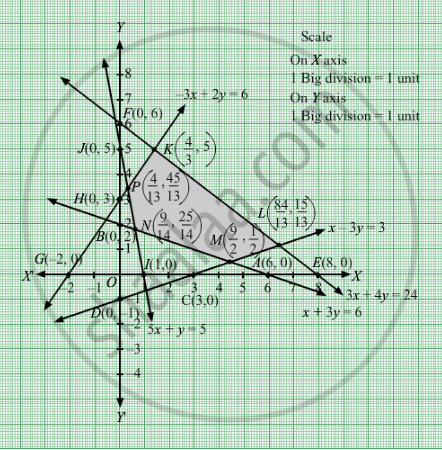# Find the Maximum and Minimum Value of 2x + Y Subject to the Constraints: X + 3y ≥ 6, X − 3y ≤ 3, 3x + 4y ≤ 24, − 3x + 2y ≤ 6, 5x + Y ≥ 5, X, Y ≥ 0. - Mathematics

Sum

Find the maximum and minimum value of 2x + y subject to the constraints:
x + 3y ≥ 6, x − 3y ≤ 3, 3x + 4y ≤ 24, − 3x + 2y ≤ 6, 5x + y ≥ 5, xy ≥ 0.

#### Solution

First, we will convert the given inequations into equations, we obtain the following equations:
x + y = 4, x + y = 3, x  2y = 2, x = 0 and y = 0.
The line x + 3y = 6 meets the coordinate axis at $A\left( 6, 0 \right)$  and B(0, 2). Join these points to obtain the line x + 3y = 6.
Clearly, (0, 0) does not satisfies the inequation x + 3y ≥ 6. So, the region in xy-plane that does not contains the origin represents the solution set of the given equation.

The line x  3y = 3 meets the coordinate axis at C(3, 0) and D(0, 1). Join these points to obtain the line x  3y = 3.
Clearly, (0, 0) satisfies the inequation x  3y ≤ 3. So, the region in xy-plane that contains the origin represents the solution set of the given equation.

The line 3x + 4y = 24 meets the coordinate axis at E(8, 0) and F(0, 6). Join these points to obtain the line 3x + 4y = 24.
Clearly, (0, 0) satisfies the inequation 3x + 4y ≤ 24. So, the region in xy-plane that contains the origin represents the solution set of the given equation.

The line 3x + 2y = 6 meets the coordinate axis at G(−2, 0) and H(0, 3). Join these points to obtain the line 3x + 2y = 6.
Clearly, (0, 0) satisfies the inequation 3x + 2y ≤ 6. So, the region in xy-plane that contains the origin represents the solution set of the given equation.

The line 5x + y = 5 meets the coordinate axis at $I\left( 1, 0 \right)$ and J(0, 5). Join these points to obtain the line 5x + y = 5.

Clearly, (0, 0) does not satisfies the inequation 5x + ≥ 5. So, the region in xy-plane that does not contains the origin represents the solution set of the given equation.

Region represented by x ≥ 0 and y ≥ 0:
Since, every point in the first quadrant satisfies these inequations. So, the first quadrant is the region represented by the inequations.
These lines are drawn using a suitable scale.The corner points of the feasible region are $\left( \frac{4}{13}, \frac{45}{13} \right)$ k $\left( \frac{4}{3}, 5 \right)$ ,L  $\left( \frac{84}{13}, \frac{15}{13} \right)$, M $\left( \frac{9}{2}, \frac{1}{2} \right)$ , N $\left( \frac{9}{14}, \frac{25}{14} \right)$ The values of Z at these corner points are as follows.

 Corner point Z = 2x + y P $\left( \frac{4}{13}, \frac{45}{13} \right)$ 2 x $\frac{4}{13}$ + $\frac{45}{13}$ = $\frac{53}{13}$ K $\left( \frac{4}{3}, 5 \right)$ 2 x $\frac{4}{3}$ + 5 = $\frac{23}{3}$ L $\left( \frac{84}{13}, \frac{15}{13} \right)$ 2 × $\frac{84}{13}$ + $\frac{15}{13}$ = $\frac{183}{13}$ M $\left( \frac{9}{2}, \frac{1}{2} \right)$ 2 × $\frac{9}{2}$ + $\frac{1}{2}$ = $\frac{19}{2}$ N $\left( \frac{9}{14}, \frac{25}{14} \right)$ 2 × $\frac{9}{14}$ + $\frac{25}{14}$ = $\frac{43}{14}$
We see that the minimum value of the objective function Z is $\frac{43}{14}$  which is at $\left( \frac{9}{14}, \frac{25}{14} \right)$ and maximum value of the objective function is $\frac{183}{13}$  which is at $\left( \frac{84}{13}, \frac{15}{13} \right)$ .
Concept: Graphical Method of Solving Linear Programming Problems
Is there an error in this question or solution?

#### APPEARS IN

RD Sharma Class 12 Maths
Chapter 30 Linear programming
Exercise 30.2 | Q 23 | Page 33